# 叠置算法(5):拓扑构建算法

1.拓扑构建的总体流程

1.1 预处理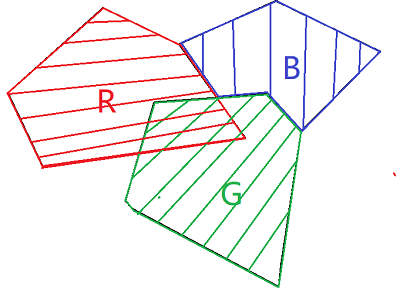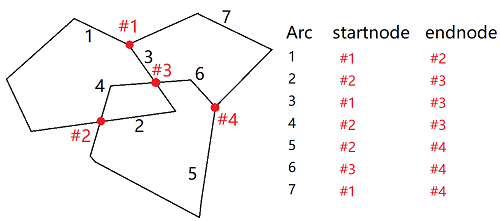1.2 拓扑构建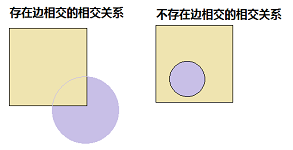。geos采用几何计算的方式获得这样的关系，考虑到geos面向二元计算效率尚可以接受，而面向数据集的空间分析运算时，构建topo face就必不可少了。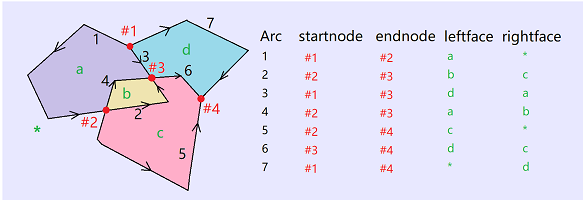在geos中，存在两套功能类似的结构，一套按照业务名称称为 geos::geomgraph ，一套按照定义称为 geos::planargraph。geos中geometry的intersection,union,difference,spatial predict 等算法都是建立在geomgraph基础上的，而一些小的算法如  operation::polygonizer 等是构建在planargraph上的。我认为这两套结构可以均合并到geomgraph中。至于为什么做两套结构，我目前还没有理解清楚。

2.拓扑构建算法

2.1 标记悬挂(dangle)：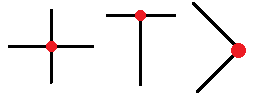节点的度数(degree)指节点关联的弧段的个数，当arc被标记为dangle时，不参与度数计算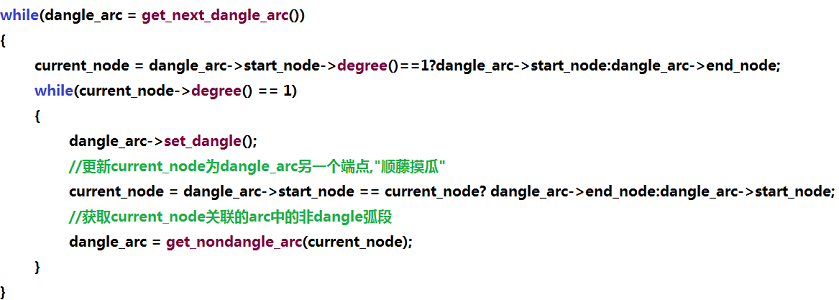2.2  拓扑构面

2.2.1 排序方法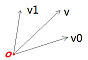1. 如果$$Quadrant(\vec v_0)<Quadrant(\vec v_1)$$ ,则$$\vec v_0<\vec v_1$$；
2. 如果$$Quadrant(\vec v_0)>Quadrant(\vec v_1)$$ ,则$$\vec v_0>\vec v_1$$；
3. 否则若$$\vec v_0\times \vec v_1>0$$;则$$\vec v_0<\vec v_1$$；

2.2.2  构面以及 cut edge的处理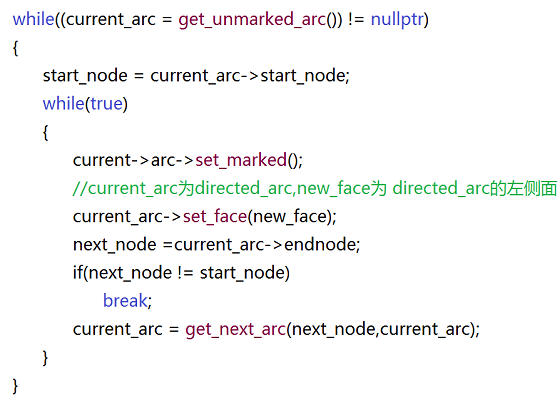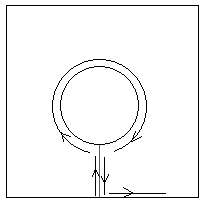2.2.3 外圈与洞的合并处理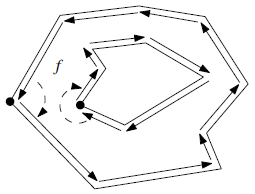1.如何判断拓扑面是逆时针顺序还是顺时针顺序。

2.如何搜索到包含内圈最内侧的外圈。

1. 首先，取内圈最左侧点（x值最小的点）$$X$$.
2. 由$$X$$向左引射线，与相交的外圈计算出x值，保留$$X$$左侧的外圈。按照交点x值由大到小排序。
3. 逐个对保留下的外圈判断点$$X$$是否在外圈内。找到的首个外圈为待求外圈。
4. 如果找不到外圈，则证明此外圈为universe face的内圈。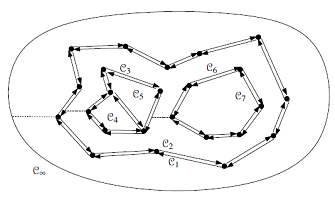3.备注

• 本文的拓扑构建方法，在部分数据结构、部分算法方面均分别参考采纳了 geos 与 《computational geometry》(第三版，Berg等著)中的部分内容。但是整体方法、流程并不完全与以上两者相同。对上两者的处理方法感兴趣的读者请注意。
• 关于程序源代码。因为叠置分析涉及的算法比较多而且复杂，因此考虑单独建立工程分享至github。目前程序正在编写中，后期会统一更新代码地址。